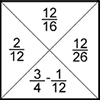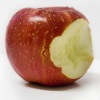# Resources tagged with: Equivalent fractions, decimals and percentages

Filter by: Content type:
Age range:
Challenge level:

### There are 9 results

Broad Topics > Fractions, Decimals, Percentages, Ratio and Proportion > Equivalent fractions, decimals and percentages### The Greedy Algorithm

##### Age 11 to 14Challenge Level

The Egyptians expressed all fractions as the sum of different unit fractions. The Greedy Algorithm might provide us with an efficient way of doing this.### Repetitiously

##### Age 14 to 16Challenge Level

Can you express every recurring decimal as a fraction?### Terminating or Not

##### Age 11 to 14Challenge Level

Is there a quick way to work out whether a fraction terminates or recurs when you write it as a decimal?### Tiny Nines

##### Age 14 to 16Challenge Level

What do you notice about these families of recurring decimals?### Fractions Jigsaw

##### Age 11 to 14Challenge Level

A jigsaw where pieces only go together if the fractions are equivalent.### Sept 03

##### Age 11 to 14Challenge Level

What is the last digit of the number 1 / 5^903 ?### Almost One

##### Age 11 to 14Challenge Level

Choose some fractions and add them together. Can you get close to 1?### Matching Fractions, Decimals and Percentages

##### Age 7 to 14Challenge Level

Can you match pairs of fractions, decimals and percentages, and beat your previous scores?### Round and Round and Round

##### Age 11 to 14Challenge Level

Where will the point stop after it has turned through 30 000 degrees? I took out my calculator and typed 30 000 ÷ 360. How did this help?